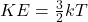## 39. When you heat a flask of water, how are you changing the ordered kinetic energy of the water molecules (if at all)? Likewis

Question

39. When you heat a flask of water, how are you changing the ordered kinetic energy of the
water molecules (if at all)? Likewise, how are you changing the random kinetic energy of the
molecules (if at all)?

in progress 0
3 months 2021-07-30T16:54:45+00:00 1 Answers 5 views 0

The average kinetic energy of the molecules increases

Explanation:

The temperature of a substance is proportional to the average kinetic energy of the particles in the substance.

In fact, for an ideal gas for instance, there is the following relationship:where

KE is the average kinetic energy of the particles

k is the Boltzmann’s constant

T is the absolute temperature of the gas

When we heat a substance (such as the flask of water in this problem), we are giving thermal energy to the particles of the substance; therefore, these particles will move faster on average, so their kinetic energy will increase (and the temperature of the substance will increase as well).